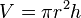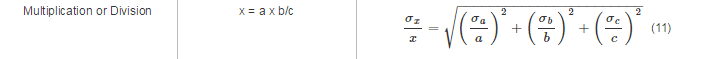# Error Propagation

## Homework Statement

You have a cylindrical tube for which you know the length is 16±0.1 cm and the radius is 8±0.1 cm, what is the error of the volume?

## Homework Equations## The Attempt at a Solution

V = π*82*16 = 3216.99 cm

But since the r term is squared, must we account for its error twice in the error propagation equation?

σV = 3216.99*√((0.1/16)2+(0.1/8)2+(0.1/8)2

Or does this require only one (0.1/8)2 term?